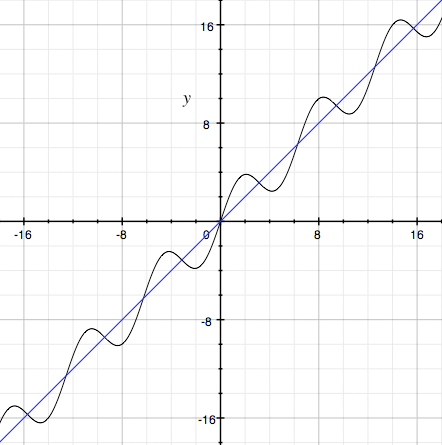SEARCH HOMEMath Central Quandaries & QueriesQuestion from amroziz, a parent: for which values of x does the graph of f(x)=x+2sinx have horizontal tangentAmroziz,

The graph of y = x is a straight lie through the origin at an angle of 45o with the positive X-axis. The graph of y = 2 sinx is periodic around the X-axis with an amplitude of 2. Hence the graph of f(x) = x + 2sinx oscillates around the line y = x. Here is its graph (the X-axis is in radians).As you can see there are infinitely many points where the graph has a horizontal tangent. To identify these points find the derivative f'(x) of f(x) with respect to x and solve f'(x) = 0. You should find two values of x with 0 ≤ x ≤ 360o. The complete solution is then these values plus or minus an integer multiple of 360o.

HarleyMath Central is supported by the University of Regina and The Pacific Institute for the Mathematical Sciences.